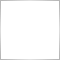how calculate divergenze241

how is the formula to calculate divergenze ?

i want to add to custom indicator the function to found divergenze

but i no found the formula to calculate it39032

asaqaz:

how is the formula to calculate divergenze ?

i want to add to custom indicator the function to found divergenze

but i no found the formula to calculate it

Search the codebase, there are several divergence indicators.241

i have already take a look anyway thanks39032

asaqaz:
i have already take a look anyway thanks
There is no "formula", you have to understand the logic and apply it to your indicator.